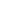New article submisson system
Submit an article in minutesOriginal research

# Prediction of heart disease using decision tree over logistic regression using machine learning with improved accuracy

## Abstract

Aim: Predicting heart disease using the Decision Tree and comparing its feature extraction precision with the Logistic Regression algorithm for improving the accuracy of the prediction. Methods and Materials: In the proposed work, predicting heart disease was carried out using machine learning algorithms such as Logistic Regression (n=10) and Decision tree (n=10). Here the pretest power analysis was carried out with 80% and the sample size for the two groups are 20. Results: From the implemented experiment, the Decision Tree accuracy significantly better than the Logistic Regression 80.10%. There is a measurable 2-tailed huge distinction in accuracy for two algorithms is 0.001 (p<0.05) Conclusion: The Decision Tree algorithm got better accuracy than Logistic Regression for Predicting heart disease.

## Imprint

K N S Shanmukha Raj, K Thinakaran. Prediction of Heart Disease using Decision Tree over Logistic Regression using Machine Learning with Improved Accuracy. Cardiometry; Issue 25; December 2022; p.1514-1519; DOI: 10.18137/cardiometry.2022.25.15141519; Available from: https://www.cardiometry.net/issues/no25-december-2022/machine-learning-improved-accuracy

## Keywords

Decision Tree,   Logistic Regression,   Principal Component Analysis,   Machine Learning,   Supervised Classification,   Novel Dimensionality ReductionCurrent issueCardiometry's libraryFounders of Cardiometry